Blog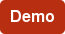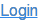# Mirus Reporter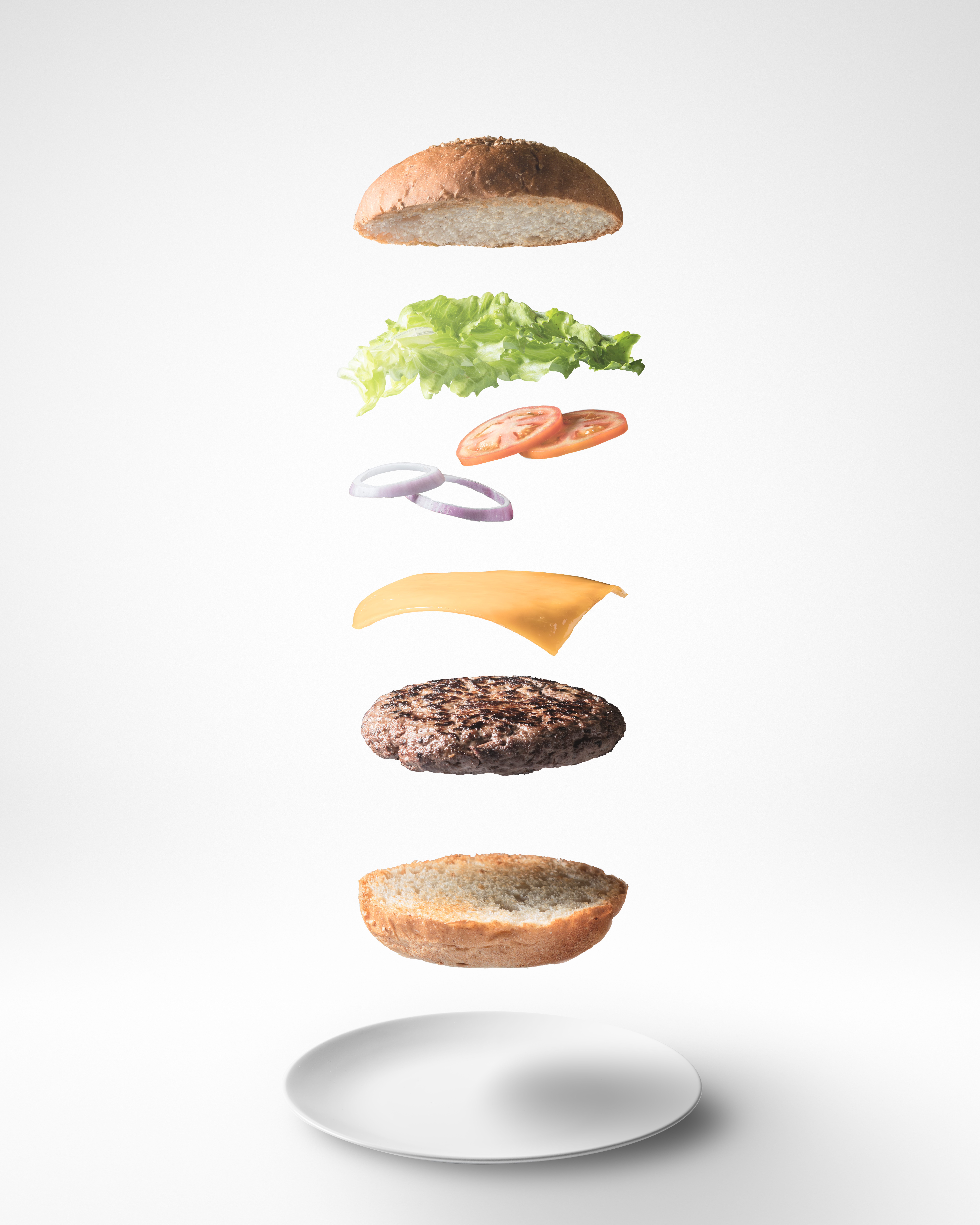You may know how much each ingredient in your restaurant costs you. But do you know how much each menu item costs? Calculating Plate Cost can help you set your menu prices, calculate inflation impact and evaluate promotion profitability. Learn how to calculate Plate Cost and download our free Plate Cost Calculator Worksheet with bonus Plate Cost Percentage calculator.

## What Is Plate Cost?

In simple terms Plate Cost is the cost of all the raw materials that go into a menu item. For this reason Plate Cost is also referred to as Menu Item Cost.

Let's take a hamburger for example. To make a hamburger you typically need:

• 1 bun
• 4 oz. Ground Beef
• 1 oz Lettuce
• 1 oz Tomato
• 3 Pickles

The ingredients listed are not everything that may be part of the hamburger. There is no mustard, mayo, or ketchup.  You can get as detailed as you like or just include the more expensive items as outlined above.

The calculation of Plate Cost uses a recipe, but it is not calculated for every restaurant using the actual price paid for each ingredient at each restaurant. Instead, you calculate Plate Cost using the average price paid during the period against a single copy of the recipes. The prices might be updated once per quarter or once a month, depending on the accuracy you determine is worthwhile. Less accuracy for significantly less cost and effort.

## How to Calculate Plate Cost

To calculate Plate Cost, first you need to know the As Served cost of each ingredient in your menu item. The As Served cost is the cost of a single serving of that item. In order to find those costs, take the cost of a product and divide it by the number of portions you make from the item.

For example, let's say you buy 80 pounds of ground beef for \$850. Since we are going to use ounces for our serving size convert the pounds to ounces for a total of 1280 ounces. Then divide the cost by the total ounces (\$850/1280) to get \$.66 per ounce. Then, since 4oz of ground beef are used in each hamburger multiply the per ounce cost (\$.66) by 4 for a total of \$2.64.

This is your As Served ingredient cost. Do this for each item individually. Each ingredient may have slightly different calculations. For example, buns are not sold by the ounce they are sold by unit.

Here is the Plate Cost for our hamburger menu item:

• 1 bun = \$.50
• 4 oz. Ground Beef = \$2.66
• 1 oz Lettuce = \$.12
• 1 oz Tomato = \$.05
• 3 Pickles = \$.02
For a total Plate Cost of \$3.34

## Plate Cost Calculator

Many, but not all, inventory management systems calculate Plate Cost. Even with a system to perform this task, the rigor of maintaining detailed, accurate recipes can be daunting, time consuming, and expensive.

Mirus clients are able to use our Dimension Maintenance tool to create Plate Cost calculations. Clients have been using this technique for decades and while it is not as accurate or up to date as a well managed perpetual inventory system, it can serve to give you an early warning that the margin of an item is beginning to erode.

In the event you do not have a back office system, aren't up for maintaining accurate recipes, or aren't a Mirus client yet a simple spreadsheet can suffice. You can make the spreadsheet as detailed as you want. The idea is to apply an 80/20 rule where you get most of the value you’d get from an inventory system without all the hassle.

We've created a Plate Cost Calculator Worksheet you can easily use to calculate Plate Cost for your menu items.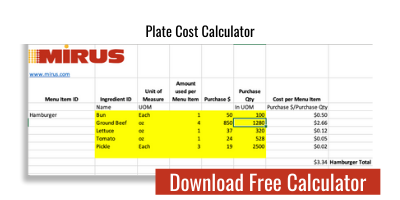To use the calculator simply enter:

• Ingredient Names
• Unit of Measure
• Amount Used Per Menu Item
• Purchase Cost
• Purchase Quantity

Here's a look at our Hamburger example plugged into the Plate Cost Calculator.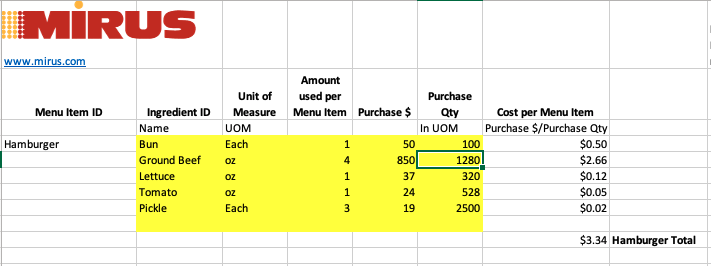The Cost per Menu Item and Total Plate Cost will be automatically calculated.

## Ways to Use Plate Cost

If you don’t have a calculation of Plate Cost for every menu item, you should. It allows you to easily monitor the cost of the basket of goods sold. It's also a simple way to evaluate whether it is time to raise prices.

You can use Plate Cost to calculate Plate Cost Percentage or Menu Item Contribution Margin. Simply divide the Plate Cost by the Menu Item Price.  The Plate Cost Calculator Worksheet has a bonus Plate Cost % Tab. Just add the Menu Item Price and the Plate Cost percentage is automatically calculated.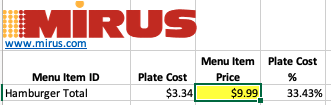Adding Plate Costs to the number of items you’ve sold will provide you with each item's profit margin/contribution margin and the total profit margin for the restaurant.

Calculating Plate Cost doesn't have to be complicated and can be very useful.